GFG App
Open AppBrowser
Continue

Scalar and Vector Quantities are used to describe the motion of an object. Scalar Quantities are defined as the physical quantities that have magnitude or size only. For example, distance, speed, mass, density, etc. However, vector quantities are those physical quantities that have both magnitude and direction like displacement, velocity, acceleration, force, mass, etc. It should be noted that when a vector quantity changes its magnitude and direction also changes similarly, when a scalar quantity changes, only its magnitude changes.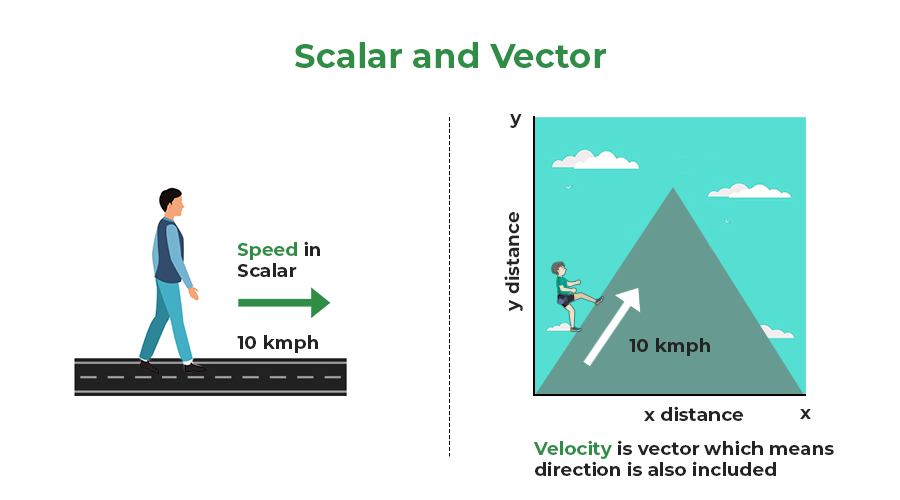## What is Scalar and Vector Quantity?

Scalar quantities are the quantities that only have a magnitude or size associated with them. These quantities can be expressed completely with a single number. Some examples of scalar quantities are – the mass of an object, the distance between two points, etc. For doing operations on scalar quantities, the usual rules of algebra work. These quantities can be added and subtracted in the same way numbers are added and subtracted. But only numbers with the same measuring unit can use the process for a scalar quantity.

### Examples of Scalar Quantities

There exist many forms of scalar quantities some of them are listed below:

• Mass
• Speed
• Distance
• Time
• Area
• Volume
• Density
• Energy
• Temperature
• Electric Charge
• Gravitational force

On the other hand, vectors are quantities that have a direction associated with them. For addition and subtraction, these quantities obey the triangle law of vector addition. Some examples of vector quantities include velocity, acceleration, etc. While describing the velocity, specifying the direction is necessary.

Any vector quantity, a can be represented in vector form as, which represents the direction and the magnitude of the vector a. In the case of the only magnitude, |a| denotes the magnitude.

### Examples of Vector Quantities

There are countless examples of vector quantities in daily life. The list of some of them is down below!

• Velocity
• Force
• Pressure
• Displacement
• Acceleration
• Thrust
• Linear momentum
• Electric field
• Polarization
• Weight

### Vector Notation

Vector notation is a way or notation used to represent a quantity that is a vector, through an arrow (⇢) above its symbol, as shown below: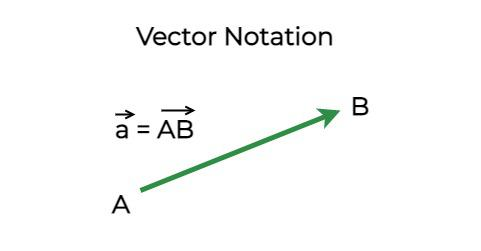## Equality of Vectors

Two vectors are considered to be equal when they have the same magnitude and same direction. The figure below shows two vectors that are equal, notice that these vectors are parallel to each other and have the same length. The second part of the figure shows two unequal vectors, which even though have the same magnitude, are not equal because they have different directions.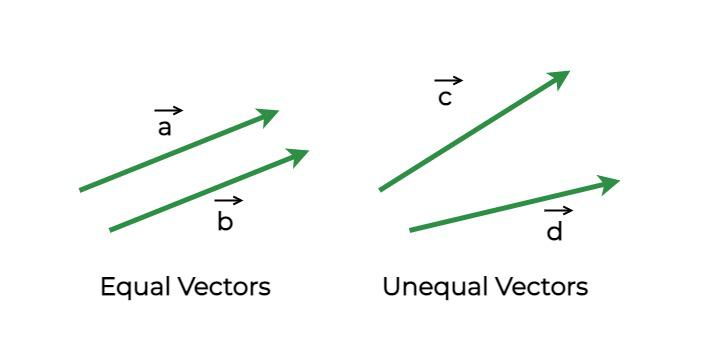## Multiplication of Vectors with Scalar

Multiplying a vector a with a constant scalar k gives a vector whose direction is the same but the magnitude is changed by a factor of k. The figure shows the vector after and before it is multiplied by the constant k. In mathematical terms, this can be rewritten as,if k > 1, the magnitude of the vector increase while it decreases when the k < 1.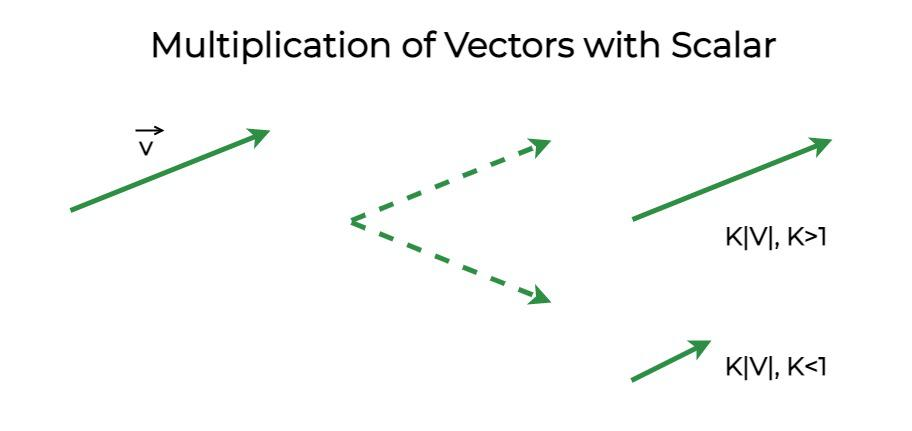Vectors cannot be added by usual algebraic rules. While adding two vectors, the magnitude and the direction of the vectors must be taken into account. Triangle law is used to add two vectors, the diagram below shows two vectors “a” and “b” and the resultant is calculated after their addition. Vector addition follows commutative property, this means that the resultant vector is independent of the order in which the two vectors are added.– (Commutative Property)

## Triangle Law of Vector Addition

Consider the vectors given in the figure above. The line PQ represents the vector “p”, and QR represents the vector “q”. The line QR represents the resultant vector. The direction of AC is from A to C.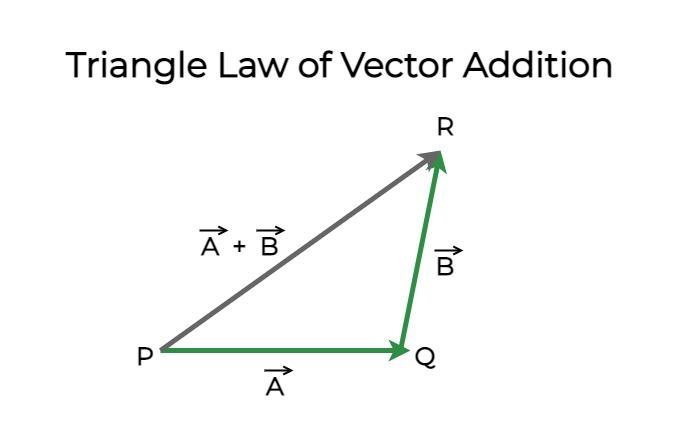Line AC represents,The magnitude of the resultant vector is given by,θ represents the angle between the two vectors. Let φ be the angle made by the resultant vector with the vector p.The above formula is known as the Triangle Law of Vector Addition.

## Parallelogram Law of Vector Addition

This law is just another way of understanding vector addition. This law states that if two vectors acting on the same point are represented by the sides of the parallelogram, then the resultant vector of these vectors is represented by the diagonals of the parallelograms. The figure below shows these two vectors represented on the side of the parallelogram.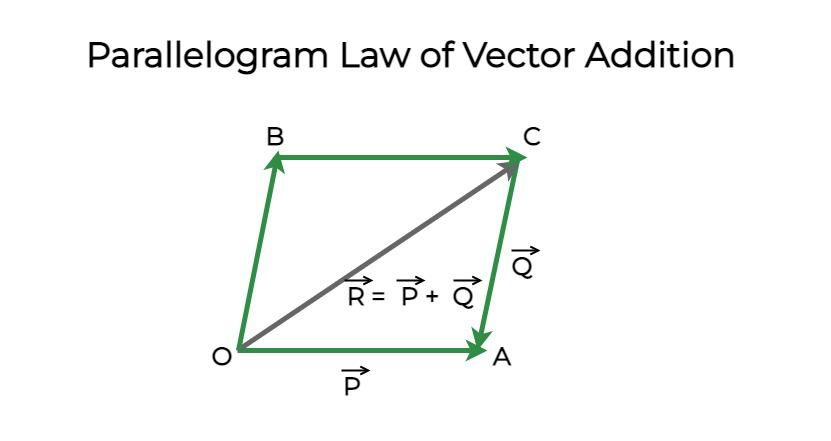## Solved Examples on Scalar and Vector

Example 1: Find the magnitude of v = i + 4j.

Solution:

|v| =a = 1, b = 4

|v| =|v| =|v| = √17

Example 2: A vector is given by, v = i + 4j. Find the magnitude of the vector when it is scaled by a constant of 5.

Solution:

|v| =5|v| = |5v|

a = 1, b = 4

|5v|

|5(i + 4j)|

|5i + 20j|

|v| =|v| =|v| = √425

Example 3: A vector is given by, v = i + j. Find the magnitude of the vector when it is scaled by a constant of 0.5.

Solution:

|v| =0.5|v| = |0.5v|

a = 1, b = 1

|0.5v|

|0.5(i + j)|

|0.5i + 0.5j|

|v| =|v| =|v| = √0.5

Example 4: Two vectors with magnitude 3 and 4. These vectors have a 90° angle between them. Find the magnitude of the resultant vectors.

Solution:

Let the two vectors be given by p and q. Then resultant vector “r” is given by,|p| = 3, |q| = 4 and|r| = 5

Example 5: Two vectors with magnitude 10 and 9. These vectors have a 60° angle between them. Find the magnitude of the resultant vectors.

Solution:

Let the two vectors be given by p and q. Then resultant vector “r” is given by,|p| = 10, |q| = 9 and## FAQs on Scalars and Vectors

Question 1: What do you mean by Scalars and Vectors, in physics?

Scalars are the physical quantities that have magnitude or size only. While vectors are the physical quantities that have both magnitude and direction.

Question 2: Give some examples of Vectors.

Here are some important examples of vectors are:

• Velocity
• Force
• Pressure
• Displacement
• Acceleration
• Thrust

Question 3: List down some important scalar quantities.

Here are some important examples of scalars are:

• Mass
• Speed
• Distance
• Time
• Area
• Volume

Question 4: Force is Scalar or Vector quantity?